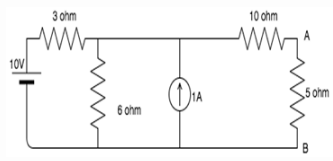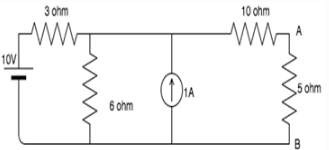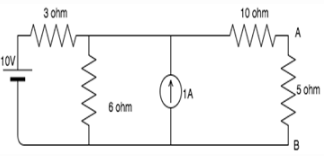# Test: Norton’s Theorem

## 10 Questions MCQ Test Network Theory (Electric Circuits) | Test: Norton’s Theorem

Description
Attempt Test: Norton’s Theorem | 10 questions in 10 minutes | Mock test for Electrical Engineering (EE) preparation | Free important questions MCQ to study Network Theory (Electric Circuits) for Electrical Engineering (EE) Exam | Download free PDF with solutions
QUESTION: 1

### The Norton current is the_______

Solution:

Norton current is the short circuit current. It is the current through the specified load resistance. It is not the open circuit current because open circuit current is equal to zero.

QUESTION: 2

### Norton resistance is found by?

Solution:

Current sources have infinite internal resistance hence behave like an open circuit whereas ideal voltage sources have 0 internal resistances hence behave as a short circuit.

QUESTION: 3

### Norton’s theorem is true for __________

Solution:

Norton’s theorem works for only linear circuit elements and not non-linear ones such as BJT, semiconductors etc.

QUESTION: 4

In Norton’s theorem Isc is__________

Solution:

Norton’s theorem states that a combination of voltage sources, current sources and resistors is equivalent to a single current source Ith and a single parallel resistor R.

QUESTION: 5

Isc is found across the ____________ terminals of the network.

Solution:

According to Norton’s theorem, Isc is found through the output terminals of a network and not the input terminals.

QUESTION: 6

Can we use Norton’s theorem on a circuit containing a BJT?

Solution:

We can use Norton’s theorem only for linear networks. BJT is a non-linear network hence we cannot apply Norton’s theorem for it.

QUESTION: 7

Calculate the Norton’s equivalent resistance for the following circuit if 5 ohm is the load resistance.​Solution:

Shorting all voltage sources and opening all current sources we have:
RN=(3||6)+10= 12 ohm.

QUESTION: 8

Calculate the short circuit current is the 5 ohm resistor is the load resistance.​Solution:

Since the 5 ohm is the load resistance, we short it and find the resistance through the short.
If we apply source transformation between the 6 ohm resistor and the 1A source, we get a 6V source in series with a 6 ohm resistor. Now we have two meshes. Let us consider I1 flowing in the first mesh and I2 flowing in the second mesh.
The mesh equations are:
9I1-6I2=4
-6I1+16I2=6
On solving these equations simultaneously, we get I2=0.72A, which is the short circuit current.

QUESTION: 9

Find the current in the 5 ohm resistance using Norton’s theorem.​Solution:

From Q8 and Q7 we have found the values of the Isc and RN respectively.
Connecting the current source in parallel to RN which is in turn connected in parallel to the load resistance=5ohm, we get the Norton’s equivalent circuit.
Using current divider: I=0.72*12/(12+5)= 0.5 A.

QUESTION: 10

Which of the following is also known as the dual of Norton’s theorem?

Solution:

Thevenin’s theorem is also known as the dual of Norton’s theorem because in Norton’s theorem we find short circuit current which is the dual of open circuit voltage-what we find in Thevenin’s theorem.Use Code STAYHOME200 and get INR 200 additional OFF Use Coupon Code